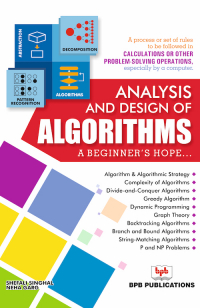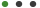Analysis and Design of Algorithms

Formats - EPUB, PDF

Pages - 220

ISBN - 9789386551894

Language - English

Published on 05/2018Description

A process or set of rules to be followed in calculations or other problem-solving operations, especially by a computer

Key Features

â— This book is especially designed for beginners, and explains all aspects of algorithm and its analysis in a simple and systematic manner.
â— Algorithms and their working are explained in detail with the help of several illustrative examples.
â— Important features like greedy algorithm, dynamic algorithm, string matching algorithm, branch and bound algorithm, NP hard and NP complete problems are suitably highlighted.
â— Solved and frequently asked questions in the various competitive examinations, sample papers of the past examinations are provided which will serve as a useful reference source.

Description

The book has been written in such a way that the concepts and working of algorithms are explained in detail, with adequate examples. To make clarity on the topic, diagrams, calculation of complexity, algorithms are given extensively throughout. Many examples are provided which are helpful in understanding the algorithms by various strategies. This content is user-focused and has been highly updated including algorithms and their real-world examples.

What will you learn

â— Algorithm & Algorithmic Strategy, Complexity of Algorithms
â— Divide-and-Conquer, Greedy, Backtracking, String-Matching Algorithm
â— Dynamic Programming, P and NP Problems
â— Graph Theory, Complexity of Algorithms

Who this book is for

The book would serve as an extremely useful text for BCA, MCA, M. Sc. (Computer Science), PGDCA, BE (Information Technology) and B. Tech. and M. Tech. students.

1. Algorithm & Algorithmic Strategy
2. Complexity of Algorithms
3. Divide-and-Conquer Algorithms
4. Greedy Algorithm
5. Dynamic Programming
6. Graph Theory
7. Backtracking Algorithms
8. Complexity of Algorithms
9. String-Matching Algorithms
10. P and NP Problems